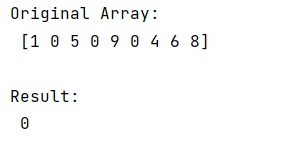# NumPy: Find first index of value fast

Learn, how to find the first index of any value in a numpy array?
Submitted by Pranit Sharma, on January 07, 2023

NumPy is an abbreviated form of Numerical Python. It is used for different types of scientific operations in python. Numpy is a vast library in python which is used for almost every kind of scientific or mathematical operation. It is itself an array which is a collection of various methods and functions for processing the arrays.

## NumPy – Finding the first index of value fast

Suppose we are given a NumPy array and we need to find the index of the first occurrence of a number in a Numpy array. Since the speed of our program is important, we need to focus on a technique that returns an output very fast.

For this purpose, we will look for the non-zero value in the NumPy array using the Bool value, it is zero then it must be False and would not return any value and if it is non-zero, it must return some value whose index would be fetched with the help of argmax() function.

Let us understand with the help of an example,

## Python code to find first index of value fast

```# Import numpy
import numpy as np

# Creating an array
arr = np.array([1,0,5,0,9,0,4,6,8])

# Display original array
print("Original Array:\n",arr,"\n")

# Finding index of a value
ind = arr.view(bool).argmax()
res = ind if arr[ind] else -1

# Display result
print("Result:\n",res,"\n")
```

Output: